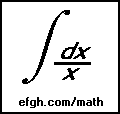#### July 4, 2001

A favorite problem in elementary probability and statistics courses is the Birthday Problem: What is the probability that at least two of N randomly selected people have the same birthday? (Same month and day, but not necessarily the same year.)

A second part of the problem: How large must N be so that the probability is greater than 50 percent? The answer is 23, which strikes most people as unreasonably small. For this reason, the problem is often called the Birthday Paradox. Some sharpies recommend betting, at even money, that there are duplicate birthdays among any group of 23 or more people. Presumably, there are some ill-informed suckers who will accept the bet.

The problem is usually simplified by assuming two things:

1. Nobody was born on February 29.
2. People's birthdays are equally distributed over the other 365 days of the year.

One of the first things to notice about this problem is that it is much easier to solve the complementary problem: What is the probability that N randomly selected people have all different birthdays? We can write this as a recursive function:

```double different_birthdays(int n)
{
return n == 1 ? 1.0 : different_birthdays(n-1) * (365.0-(n-1))/365.0;
}
```
Obviously, for N = 1 the probability is 1. For N>1, the probability is the product of two probabilities:
1. That the first N-1 people have all different birthdays.
2. That the N-th person has a birthday different from any of the first N-1.

A program to display the probabilities goes something like this:

```void main(void)
{
int n;
for (n = 1; n <= 365; n++)
printf("%3d: %e\n", n, 1.0-different_birthdays(n));
}
```
The result is something like this:
```  1: 0.000000e+00
2: 2.739726e-03
3: 8.204166e-03
4: 1.635591e-02
5: 2.713557e-02
***
20: 4.114384e-01
21: 4.436883e-01
22: 4.756953e-01
23: 5.072972e-01
24: 5.383443e-01
25: 5.686997e-01
***
```

The probability that at least two of N people have the same birthday rises above 0.5 when N=23.

The original problem can be solved with a slide rule, which is exactly what I did when I first heard it many, many years ago.

If we add February 29 to the mix, it gets considerably more complicated. In this case, we make some additional assumptions:

1. Equal numbers of people are born on days other than February 29.
2. The number of people born on February 29 is one-fourth of the number of people born on any other day.

Hence the probability that a randomly selected person was born on February 29 is 0.25/365.25, and the probability that a randomly selected person was born on another specified day is 1/365.25.

The probability that N persons, possibly including one born on February 29, have distinct birthdays is the sum of two probabilities:

1. That the N persons were born on N different days other than February 29.
2. That the N persons were born on N different days, and include one person born on February 29.

The probabilities add because the two cases are mutually exclusive.

Now each probability can be expressed recursively:

```double different_birthdays_excluding_Feb_29(int n)
{
return n == 1 ? 365.0/365.25  :
different_birthdays_excluding_Feb_29(n-1) * (365.0-(n-1)) / 365.25;
}

double different_birthdays_including_Feb_29(int n)
{
return n == 1 ? 0.25 / 365.25 :
different_birthdays_including_Feb_29(n-1) * (365.0-(n-2)) / 365.25 +
different_birthdays_excluding_Feb_29(n-1) * 0.25 / 365.25;
}
```

A program to display the probabilities goes something like this:

```void main(void)
{
int n;
for (n = 1; n <= 366; n++)
printf("%3d: %e\n", n, 1.0-different_birthdays_excluding_Feb_29(n) -
different_birthdays_including_Feb_29(n));
}
```

The result is something like this:

```  1: -8.348357e-18
2: 2.736445e-03
3: 8.194354e-03
4: 1.633640e-02
5: 2.710333e-02
***
20: 4.110536e-01
21: 4.432853e-01
22: 4.752764e-01
23: 5.068650e-01
24: 5.379013e-01
25: 5.682487e-01
***
```

As expected, the probabilities are slightly lower, because there is a lower probability of matching birthdays when there are more possible birthdays. But the smallest number with probability greater than 0.5 is still 23.

Of course, a mathematical purist may argue that leap years don't always come every four years, so the calculations need further modification. However, the last quadrennial year that wasn't a leap year was 1900, and the next one will be 2100. The number of persons now living who were born in 1900 is so small that I think our approximation is valid for all practical purposes. But you are welcome to make the required modifications if you wish.

The Birthday Paradox has implications beyond the world of parlor betting. A standard technique in data storage is to assign each item a number called a hash code. The item is then stored in a bin corresponding to its hash code. This speeds up retrieval because only a single bin must be searched. The Birthday Paradox shows that the probability that two or more items will end up in the same bin is high even if the number of items is considerably less than the number of bins. Hence efficient handling of bins containing two or more items is required in all cases.